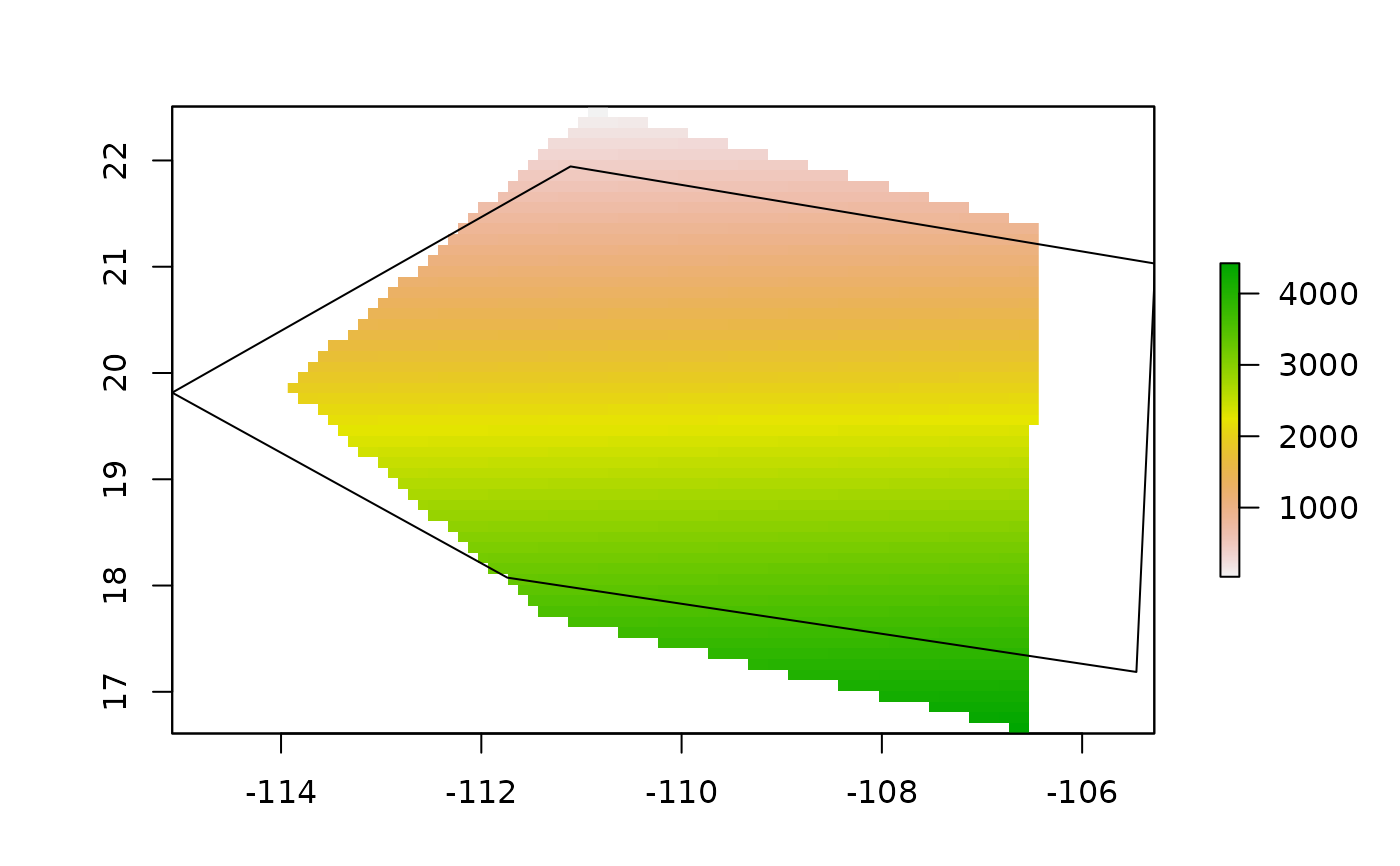shape2Grid creates a raster grid given a spatial polygon and a grid resolution.

## Usage

shape2Grid(shpolygon, resolution, ones = TRUE)

## Arguments

shpolygon

A SpatialPolygon, SpatialPolygonDataFrame representing the desired shape of the grid.

resolution

Numeric. Spatial resolution of the grid.

ones

Logical. Fill with ones the values of the raster. If not the values will be written as cellID values.

## Value

Returns a raster object with the shape of 'shpolygon' of a given resolution.

## Author

Luis Osorio-Olvera & Jorge Soberón

## Examples

x_coord <- c(-106.5699, -111.3737,-113.9332, -110.8913, -106.4262, -106.5699)
y_coord <- c(16.62661, 17.72373, 19.87618, 22.50763, 21.37728, 16.62661)
xy <- cbind(x_coord, y_coord)
p <- sp::Polygon(xy)
ps <- sp::Polygons(list(p),1)
sps <- sp::SpatialPolygons(list(ps))
r1 <- bamm::shape2Grid(sps,resolution = 0.1,ones = FALSE)
plot(r1)